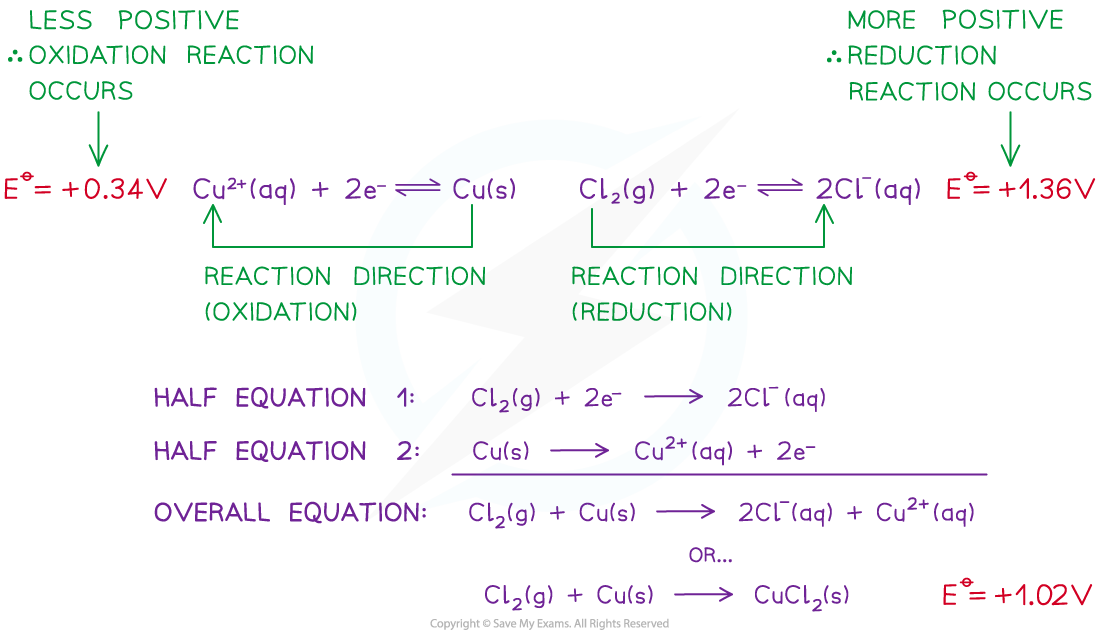# AQA A Level Chemistry复习笔记5.4.3 Predicting Reactions

### Predicting Reactions

#### Direction of electron flow

• The direction of electron flow can be determined by comparing the Eꝋ values of two half-cells in an electrochemical cell

Cl2 (g) + 2e- ⇌ 2Cl- (aq)        Eꝋ = +1.36 V

Cu2+ (aq) + 2e- ⇌ Cu (s)        Eꝋ = +0.34 V

• The Cl2 more readily accept electrons from the Cu2+/Cu half-cell
• This is the positive pole
• Cl2 gets more readily reduced

• The Cu2+ more readily loses electrons to the Cl2/Cl- half-cell
• This is the negative pole
• Cu2+ gets more readily oxidised

• The electrons flow from the Cu2+/Cu half-cell to the Cl2/Cl- half-cell
• The flow of electrons is from the negative pole to the positive poleThe electrons flow through the wires from the negative pole to the positive pole

#### Feasibility

• The Eꝋ values of a species indicate how easily they can get oxidised or reduced
• The more positive the value, the easier it is to reduce the species on the left of the half-equation
• The reaction will tend to proceed in the forward direction

• The less positive the value, the easier it is to oxidise the species on the right of the half-equation
• The reaction will tend to proceed in the backward direction
• A reaction is feasible (likely to occur) when the Ecellꝋ is positive

• For example, two half-cells in the following electrochemical cell are:

Cl2 (g) + 2e- ⇌ 2Cl- (aq)        Eꝋ = +1.36 V

Cu2+ (aq) + 2e- ⇌ Cu (s)        Eꝋ = +0.34 V

• Cl2 molecules are reduced as they have a more positive Eꝋ value
• The chemical reaction that occurs in this half cell is:

Cl2 (g) + 2e- → 2Cl- (aq)

• Cu2+ ions are oxidised as they have a less positive Eꝋ value
• The chemical reaction that occurs in this half cell is:

Cu (s) → Cu2+ (aq) + 2e-

• The overall equation of the electrochemical cell is (after cancelling out the electrons):

Cu (s) + Cl2 (g) → 2Cl- (aq) + Cu2+ (aq)

OR

Cu (s) + Cl2 (g) → CuCl2 (s)

• The forward reaction is feasible (spontaneous) as it has a positive Eꝋ  value of +1.02 V ((+1.36) - (+0.34))
• The backward reaction is not feasible (not spontaneous) as it has a negative Eꝋ value of -1.02 ((+0.34) - (+1.36))A reaction is feasible when the standard cell potential Eꝋ is positive

#### Exam Tip

Remember that the electrons only move through the wires in the external circuit and not through the electrolyte solution.